# 基于K邻近算法的城市道路短时交通预测Urban Short-Term Traffic Prediction Based on K-Nearest Neighbor Method

DOI: 10.12677/HJDM.2018.84, PDF, HTML, XML, 下载: 742  浏览: 1,848

Abstract: To improve the accuracy of urban traffic prediction, a framework based on K-Nearest Neighbor (KNN) methods is proposed to adapt to drastic changes in traffic conditions. The eigenvector con-sists of the time series of average speed and the difference series considering the changes on the real urban traffic. Arolling horizon approach is proposed to predict the short-term traffic of different urban road sections. The empirical results of Shenzhen presented that the KNN method with the difference series is able to achieve a short-term and real-time prediction for different urban road sections. On the comparisons with Support Vector Machine and Random Forest, the KNN is more suitable to the prediction of sub-arterial road with highly variable traffic state.

1. 引言

2. 基于KNN算法的预测框架构建

2.1. KNN算法

KNN算法是数据挖掘技术中的分类方法之一，基于实例学习的非参数预测思想，通过搜索历史数据库中与待预测特征向量最相似的K个记录来进行分类。如果一个样本在特征空间中K个最相似(即特征空间中最近邻)的样本中的大多数属于某一个类别，则该样本也属于这个类别。换句话说，KNN算法中所选择的近邻样本都是已经正确分类的对象，并只依据最邻近的一个或者几个样本的类别来决定待分样本所属的类别。如图1所示，在某一特征空间存在w1，w2，w3三个分类，通过计算某一样本X的所有历史样本的度量距离，寻找K个最邻近历史样本。图中样本X的5个最邻近历史样本中，有3个从属于分类w1，有2个从属于分类w3，因此确定样本X从属于分类w1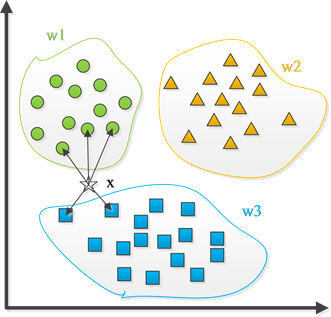Figure 1. The classification diagram of KNN method

2.2. 基于KNN算法的预测框架

2.2.1. 数据预处理

KNN算法的预测精确度很大程度上由历史数据集的质量决定，一个数据充沛、完善、采样率合适的历史数据集是KNN准确预测的前提条件。本文采用出租汽车GPS (Global Positioning System)、百度导航与公交车GPS三者融合得到的速度结果数据，数据分辨率为5分钟。总体数据样本较充足，但存在以下三个问题：

 历史数据量大导致预测计算量大；

 部分路段存在某些时间片数据缺失；

 少数偏远区域的路段数据样本量严重不足。

2.2.2. 确定特征向量

KNN算法的特征向量，不仅需要与待预测的交通状态具有较强的相关性，能反映历史数据集合中的个体间差异，同时在预测过程中还需要及时从实时数据中获取以满足在线短时预测的要求。由于待预测的平均速度具有时间序列自相关性，因此本文选择平均速度的时间序列作为当前时间点的特征向量，即

${V}_{t}^{eig}=\left[{v}_{t-1},{v}_{t-2},\cdots ,{v}_{t-n}\right]$ (1)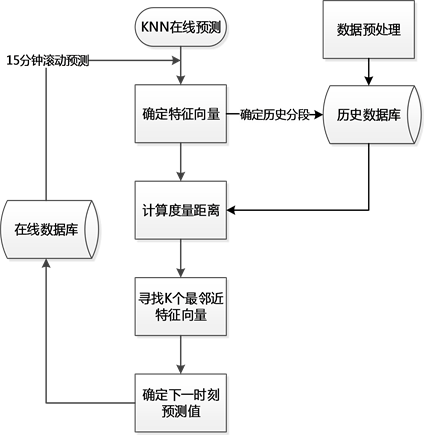Figure 2. The prediction framework of KNN method

${V}_{t}^{diff}=\left[{d}_{t-1},{d}_{t-2}\right]=\left[{v}_{t-1}-{v}_{t-2},{v}_{t-2}-{v}_{t-3}\right]$ (2)

${V}_{t}^{eig}=\left[{v}_{t-1},{v}_{t-2},{v}_{t-3},{V}_{t}^{diff}\right]$ (3)

2.2.3. 历史数据分段

2.2.4. 确定距离度量

$d\left({V}_{t}^{eig},{H}_{a}^{eig}\right)=|{V}_{t}^{eig}-{H}_{a}^{eig}|=\sqrt{\underset{n=1}{\overset{3}{\sum }}{w}_{n}{\left({v}_{t-n}-{h}_{a-n}\right)}^{2}+\underset{n=1}{\overset{2}{\sum }}{\left({d}_{t-n}-{q}_{a-n}\right)}^{2}},\text{\hspace{0.17em}}\text{\hspace{0.17em}}a\in \left[1,N\right]$ (4)Figure 3. The historical data subsection of KNN prediction

2.2.5. K值的标定

K值是KNN算法中的唯一参数，其取值直接影响模型预测的结果。不同道路类型、不同时段实现精确预测的K值是不相同的。因此，本文采用交叉验证的方法进行K值的标定，具体步骤如下所示：

${E}_{t}^{\text{MAPE}}=|\frac{{v}_{t}^{obs}-{v}_{t}^{pre}}{{v}_{t}^{obs}}|\ast 100%$ (5)

${M}_{K}^{\text{MAPE}}=\frac{1}{N}\underset{n=1}{\overset{N}{\sum }}{E}_{n}^{\text{MAPE}}$ (6)

2.2.6. 确定下一时刻预测值

${v}_{t}^{pre}=\underset{n=1}{\overset{K}{\sum }}{u}_{n}{v}_{t}^{his}$ (7)

${u}_{n}=\frac{\mathrm{exp}\left(-d\left({V}_{t}^{eig},{H}_{n}^{eig}\right)\right)}{\underset{n=1}{\overset{K}{\sum }}\mathrm{exp}\left(-d\left({V}_{t}^{eig},{H}_{n}^{eig}\right)\right)}$ (8)

2.2.7. 滚动预测

3. 实例验证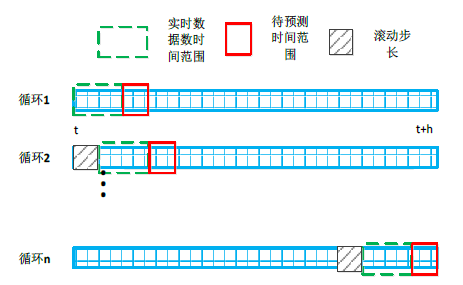(a)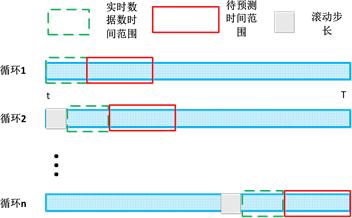(b)

Figure 4. The double-loop rolling prediction of KNN method. (a) Is the first rolling; (b) Is the second rollingTable 1. The basic information of typical road section

3.1. K值标定

K的最小值设定为1，最大值设定为50。各条典型路段的标定结果如图5所示。

3.2. 所有路段预测结果

3.3. 特征向量的比较

3.3.1. 高速路与快速路

7月24日(周日)，高速路与快速路在前段时间维持较高的预测精度。然而，高速路的预测误差突然随着路段平均速度的骤降急剧增大，原因在于路段发生了交通事故，对应时段的历史数据集中没有对应的历史交通状态信息。但是，增加差分序列后，KNN能够在这时间段内很好地识别出当前交通状态的急剧变化，及时调整预测值，尽可能提高预测精确度。快速路同样在晚间平均速度下降时预测误差稍微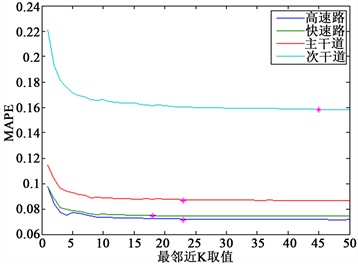Figure 5. The calibration results of tipycal road section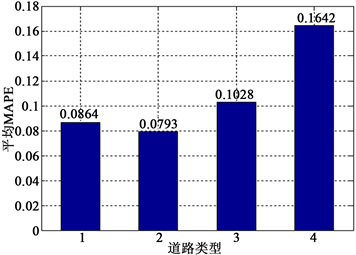Figure 6. The prediction hisrogram of MAPETable 2. The KNN prediction MAPE of different types of road sections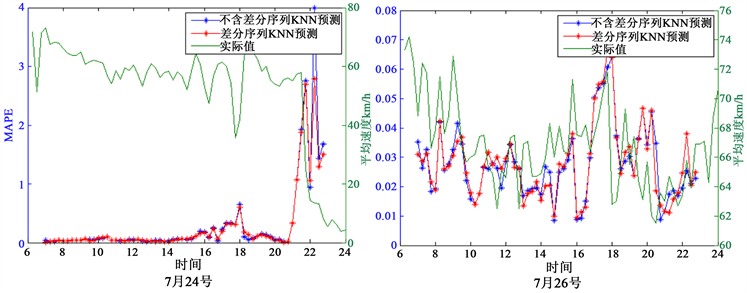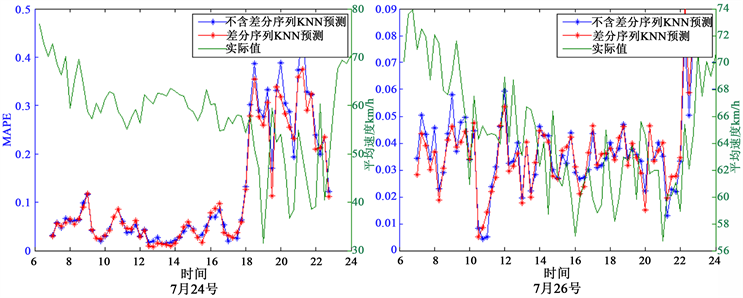Figure 7. The prediction line chart of freeway (up) and expressway (down)

3.3.2. 主干路与次干路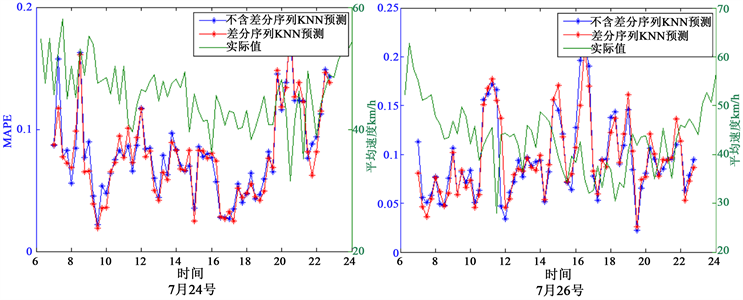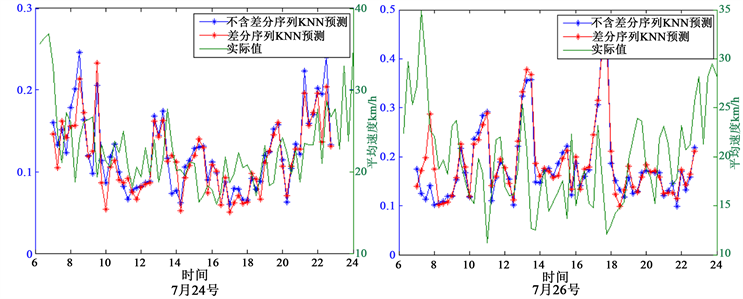Figure 8. The prediction line chart of arterial road (up) and sub-arterial road (down)

3.4. 与其他预测算法的对比

4. 总结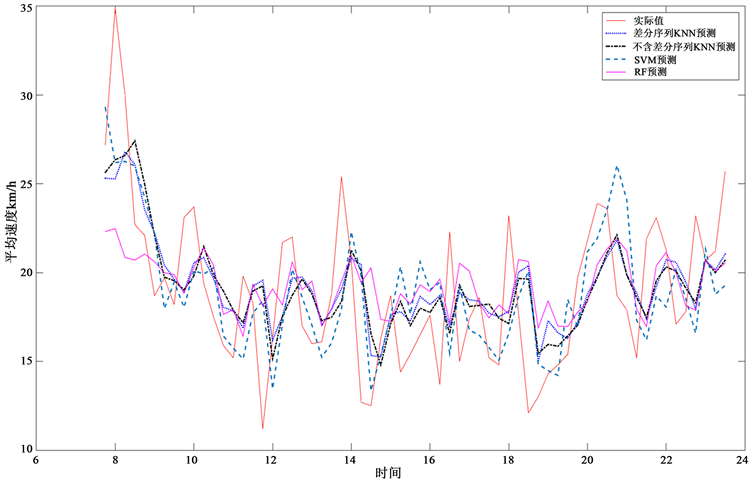Figure 9. The prediction line chart of different algorithms

 高速路的交通状态变化平稳，KNN的预测结果最为准确。但是，当高速路发生交通事故时，如果历史数据库中没有含有足够的相似信息，KNN的预测精度会急剧下降，这可作为发生交通事故的预警信息，这也是本文下一阶段的研究内容之一。

 快速路与主干路的交通状态呈现较为明显的早晚高峰的特征，历史数据库中包含足够的特征信息，KNN的预测值与实际值的误差较小。此外，交通状态的变化幅度也是影响KNN预测精度的一个重要因素之一。交通状态变化幅度越大，KNN预测框架的预测精度越低。作为特征向量的补充向量，差分序列能够很好地增强KNN捕捉交通变化的能力，提高预测的精确度。

 针对交通状态变化幅度最大的次干路，KNN算法能够及时跟上变化的交通状态，比SVM和RF预测算法获得更准确的预测结果，且差分序列是提高KNN预测精度的有效特征向量之一。

NOTES

*通讯作者。

  王晓原, 刘海红, 王凤群, 王晓辉. 交通流短时预测理论研究进展[J]. 交通标准化, 2006(12): 156-158.  王进, 史其信. 短时交通流预测模型综述[J]. 中国公共安全, 2005, 6(1): 92-98.  蔡岩. 基于灰色预测模型的短期交通流预测研究[D]: [硕士学位论文]. 成都: 西南交通大学, 2009.  钱晓东, 王正欧. 基于改进KNN的文本分类方法[J]. 情报科学, 2005, 23(4): 550-554.  陈婧敏. 基于KNN回归的短时交通流预测[J]. 微型电脑应用, 2015, 31(9): 25-29.  王翔, 陈小鸿, 杨祥妹. 基于K最近邻算法的高速公路短时行程时间预测[J]. 中国公路学报, 2015, 28(1): 102-111.  Myung, J., Kim, D., Kho, S., et al. (2011) Travel Time Prediction Uding K Nearest Neighbor Method with Combined Data from Vehicle Detector System and Automatic Toll Collection System. Transportation Research Record, 2256, 51-59. https://doi.org/10.3141/2256-07  Bustillos, B. and Chui, Y. (2011) Real Time Freeway Experienced Travel Time Prediction Using N-Curve and K Nearest Neighbor Methods. Transportation Research Record, 2243, 127-137. https://doi.org/10.3141/2243-15  Pan, T., Sumalee, A., Zhong, R. and Indra-Payoong, N. (2013) Short-Term Traffic State Prediction Based on Temporal-Spatial Correlation. IEEE Transactions on Intelligent Transportation Systems, 14, 1242-1254. https://doi.org/10.1109/TITS.2013.2258916  Sumalee, A., Pan, T., Zhong, R. and Uno, N. (2013) Dynamic Sto-chastic Journey Time Estimation and Reliability Analysis Using Stochastic Cell Transmission Model: Algorithm and Case Studies. Transportation Research Part C, 35, 263-285. https://doi.org/10.1016/j.trc.2012.11.003